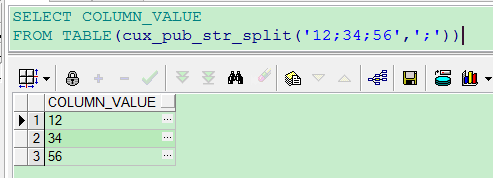oracle 两个逗号分割的字符串 如何判断是否其中有相同值

3个回答

oracle 取交集函数intersect
select regexp_substr(nme, '[^,]+', 1, rownum) nme
from (select '北京,杭州,上海' nme from dual)
connect by rownum <= length(regexp_replace(nme, '[^,]+')) +1
intersect
select regexp_substr(nme, '[^,]+', 1, rownum) nme
from (select '北京,上海,四川,郑州' nme from dual)
connect by rownum <= length(regexp_replace(nme, '[^,]+')) +1;

1.CREATE OR REPLACE TYPE ty_str_split IS TABLE OF VARCHAR2 (4000);
2.CREATE OR REPLACE FUNCTION cux_pub_str_split (p_str IN VARCHAR2, p_delimiter IN VARCHAR2)
RETURN ty_str_split PIPELINED
IS
j INT := 0;
i INT := 1;
len INT := 0;
len1 INT := 0;
str VARCHAR2 (4000);
BEGIN
len := LENGTH (p_str);
len1 := LENGTH (p_delimiter);
WHILE j < len
LOOP
j := INSTR (p_str, p_delimiter, i);
IF j = 0
THEN
j := len;
str := SUBSTR (p_str, i);
PIPE ROW (str);
IF i >= len
THEN
EXIT;
END IF;
ELSE
str := SUBSTR (p_str, i, j - i);
i := j + len1;
PIPE ROW (str);
END IF;
END LOOP;
RETURN;
END cux_pub_str_split;

3.测试数据，可以转化成表，然后用表的形式进行判断